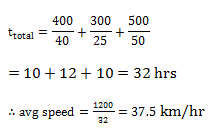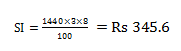# SSC Quantitative Aptitude Questions (Day-75)

Dear Aspirants, you can find the Quantitative Aptitude questions with detailed explanations for the SSC exams. Nowadays the competitive level of the exam has been increasing consistently. Due to the great demand for the government job, the level of the toughness reached greater. Candidates have to enhance the preparation process in order to drive in the right path. It doesn’t need to clear the prescribed cutoff. You must have to score good marks more than the cut off marks to get into the final provisional list. Here we have updating the Quantitative Aptitude questions with detailed explanations on a daily basis. You can practice with us and measure your level of preparation. According to that you can sculpt yourself in a proper way. SSC aspirants kindly make use of it and grab your success in your career.

Start Quiz

1) 50 litres of mixture of alcohol and water is in the ratio of 1 : 4 respectively. If 10 litres of mixture is taken off and replaced with 10 litres of water. Then what is the new ratio of alcohol and water respectively?

(a) 1 : 4

(b) 2 : 11

(c) 1 : 5

(d) 4 : 21

2) Average of 7 consecutive odd numbers is 31. If the previous and next odd number to these 7 odd numbers are also included, then what will be the new average?

(a) 33

(b) 31

(c) 35

(d) 29

3) Average weight of a class of boys is 24 kg. When a boy having weight 36 kg leaves the class and a new boy having weight 30 kg joins the class, then the average weight become 23.5 kg. How many boys are there in the class?

(a) 12

(b) 8

(c) 6

(d) 16

4) An article is sold at a profit of 50%. If the cost price is doubled and the selling price is halved, then what will be the new profit or loss percentage?

(a) 62.5 Loss

(b) 62.5 Profit

(c) 37.5 Loss

(d) 37.5 Profit

5) By selling a pen for Rs 105 there is a loss of 30%. At what price (in Rs) the pen must be sold in order to gain 30%?

(a) 215

(b) 165

(c) 195

(d) 200

6) Population of a town increases by 12% every year. If the population of town will be 188160 after 2 years, then what is its present population?

(a) 150000

(b) 160000

(c) 155000

(d) 165000

7) A 120 litres mixture of milk and water contains 40% milk. How much milk (in litres) must be added so that milk becomes 50%?

(a) 28

(b) 30

(c) 24

(d) 32

8) A person goes to his office by scooter at the speed of 50 km/hr and reaches 10 minutes earlier. If he goes at the speed 40 km/hr, then he reaches 20 minutes late. What will be the speed (in km/hr) of the scooter to reach on time?

(a) 47 4/13

(b) 46 2/13

(c) 48 2/13

(d) 47 2/13

9) One third of a certain journey is covered at the speed of 40 km/hr, one fourth at the speed of 25 km/hr and the rest at the speed of 50 km/hr. what will be the average speed(in km/hr) for the whole journey?

(a) 37.5

(b) 33.5

(c) 33.33

(d) 38.33

10) What is the simple interest (in Rs) on a principle sum of Rs 1440 for 3 years at the rate of 8% per annum?

(a) 345.6

(b) 230.4

(c) 367.8

(d) 312.2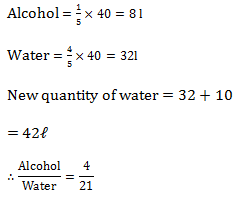a – 6, a – 4, a- 2, a , a + 2, a + 4, a + 6

∴ a = 31

New average = 9a/9 = a= 31

∑boys = 24b

∑ boys – 36 + 30 = 23.5b

24b – 6 = 23.5b

Or 0.5b = 6

Or, b = 12

Let CP = 100

∴ SP = 150

Now, SP = 75 & CP = 200

So, Loss = 125

Loss% = 125/200 × 100 = 62.5%

105 = 0.7CP

Or, CP = 150

So, SP =1.3CP = 1.3 × 150

= 130 + 65

= Rs 195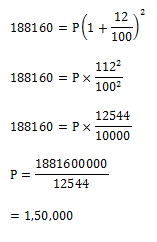Milk = 0.4 × 120

= 48ℓ

Water = 72 ℓ

Quantity of water remaining same

∴ milk = 72ℓ

So, quantity to be added = 72 – 48 = 24 ℓ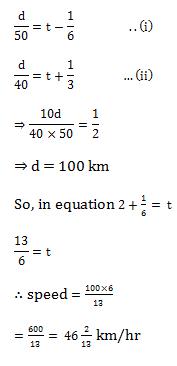Let distance be 1200 km

∴ 400 km at 40 km/hr

300 km at 25 km/hr

& 500 km at 50 km/hr

So,